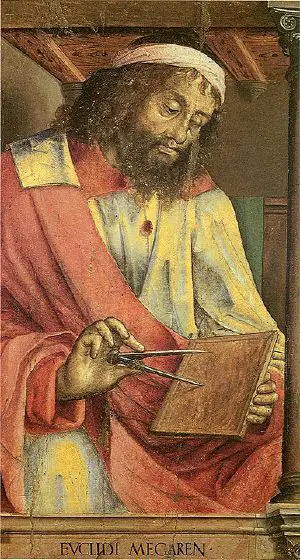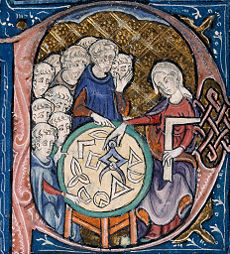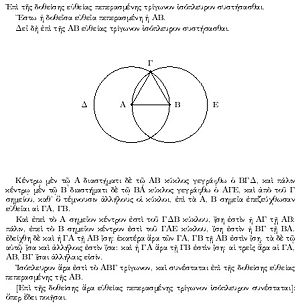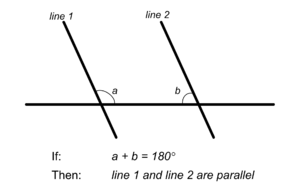# EuclidEuclid as depicted by Justus van Ghent, c. fifteenth-century; no likeness or description of Euclid's physical appearance made during his lifetime survives

Euclid (also referred to as Euclid of Alexandria) (Greek: Εὐκλείδης) (c. 325 B.C.E. – c. 265 B.C.E.), a Greek mathematician, who lived in Alexandria, Hellenistic Egypt, almost certainly during the reign of Ptolemy I (323 B.C.E.–283 B.C.E.), is often referred to as the "father of geometry." His most popular work, Elements, is thought to be one of the most successful textbooks in the history of mathematics. Within it, the properties of geometrical objects are deduced from a small set of axioms, establishing the axiomatic method of mathematics. Euclid thus imposed a logical organization on known mathematical truths, by the disciplined use of logic. Later philosophers adapted this methodology to their own fields.

Although best-known for its exposition of geometry, the Elements also includes various results in number theory, such as the connection between perfect numbers and Mersenne primes, the proof of the infinitude of prime numbers, Euclid's lemma on factorization (which lead to the fundamental theorem of arithmetic, on uniqueness of prime factorizations), and the Euclidean algorithm for finding the greatest common divisor of two numbers. Elements was published in approximately one thousand editions, and was used as the basic text for geometry by the Western world for two thousand years.

Euclid also wrote works on perspective, conic sections, spherical geometry, and possibly quadric surfaces. Neither the year nor place of his birth have been established, nor the circumstances of his death.

## Life

Little is known about Euclid outside of what is presented in Elements and his other surviving books. What little biographical information we do have comes largely from commentaries by Proclus and Pappus of Alexandria: Euclid was active at the great Library of Alexandria and may have studied at Plato's Academy in Greece. Euclid's exact lifespan and place of birth are unknown. Some writers in the Middle Ages erroneously confused him with Euclid of Megara, a Greek Socratic philosopher who lived approximately one century earlier.

## Works

Euclid’s most famous work, Elements, is thought to be one of the most successful textbooks in the history of mathematics. Within it, the properties of geometrical objects are deduced from a small set of axioms, establishing the axiomatic method of mathematics.

In addition to the Elements, five works of Euclid have survived to the present day.

• Data deals with the nature and implications of "given" information in geometrical problems; the subject matter is closely related to the first four books of the Elements.
• On Divisions of Figures, which survives only partially in Arabic translation, concerns the division of geometrical figures into two or more equal parts or into parts in given ratios. It is similar to a third-century C.E. work by Heron of Alexandria, except that Euclid's work characteristically lacks any numerical calculations.
• Phaenomena concerns the application of spherical geometry to problems of astronomy.
• Optics, the earliest surviving Greek treatise on perspective, contains propositions on the apparent sizes and shapes of objects viewed from different distances and angles.
• Catoptrics, which concerns the mathematical theory of mirrors, particularly the images formed in plane and spherical concave mirrors.

All of these works follow the basic logical structure of the Elements, containing definitions and proved propositions.

There are four works credibly attributed to Euclid which have been lost.

• Conics was a work on conic sections that was later extended by Apollonius of Perga into his famous work on the subject.
• Porisms might have been an outgrowth of Euclid's work with conic sections, but the exact meaning of the title is controversial.
• Pseudaria, or Book of Fallacies, was an elementary text about errors in reasoning.
• Surface Loci concerned either loci (sets of points) on surfaces or loci which were themselves surfaces; under the latter interpretation, it has been hypothesized that the work might have dealt with quadric surfaces.

## Elements

Euclid's Elements (Greek: Στοιχεῖα) is a mathematical and geometric treatise, consisting of thirteen books, written around 300 B.C.E. It comprises a collection of definitions, postulates (axioms), propositions (theorems and constructions), and proofs of the theorems. The thirteen books cover Euclidean geometry and the ancient Greek version of elementary number theory. The Elements is the oldest extant axiomatic deductive treatment of mathematics, and has proven instrumental in the development of logic and modern science.

Euclid's Elements is the most successful textbook ever written. It was one of the very first works to be printed after the printing press was invented, and is second only to the Bible in number of editions published (well over one thousand). It was used as the basic text on geometry throughout the Western world for about two thousand years. For centuries, when the quadrivium was included in the curriculum of all university students, knowledge of at least part of Euclid's Elements was required of all students. Not until the twentieth century did it cease to be considered something all educated people had read.

The geometrical system described in Elements was long known simply as "the" geometry. Today, however, it is often referred to as Euclidean geometry to distinguish it from other so-called non-Euclidean geometries which were discovered during the nineteenth century. These new geometries grew out of more than two millennia of investigation into Euclid's fifth postulate (Parallel postulate), one of the most-studied axioms in all of mathematics. Most of these investigations involved attempts to prove the relatively complex and presumably non-intuitive fifth postulate using the other four (a feat which, if successful, would have shown the postulate to be in fact a theorem).

### HistoryWoman teaching geometry, illustration at the beginning of a medieval translation of Euclid's Elements (c. 1310)

Scholars believe that Elements is largely a collection of theorems proved by earlier mathematicians in addition to some original work by Euclid. Euclid’s text provides some missing proofs, and includes sections on number theory and three-dimensional geometry. Euclid's famous proof of the infinitude of prime numbers is in Book IX, Proposition 20.

Proclus, a Greek mathematician who lived several centuries after Euclid, writes in his commentary of the Elements: "Euclid, who put together the Elements, collecting many of Eudoxus's theorems, perfecting many of Theaetetus's, and also bringing to irrefragable demonstration the things which were only somewhat loosely proved by his predecessors."

A version by a pupil of Euclid called Proclo was translated later into Arabic after being obtained by the Arabs from Byzantium and from those secondary translations into Latin. The first printed edition appeared in 1482 (based on Giovanni Campano’s 1260 edition), and since then it has been translated into many languages and published in approximately one thousand different editions. In 1570, John Dee provided a widely respected "Mathematical Preface," along with copious notes and supplementary material, to the first English edition by Henry Billingsley.

Copies of the Greek text also exist in the Vatican Library and the Bodlean Library in Oxford. However, the manuscripts available are of very variable quality and invariably incomplete. By careful analysis of the translations and originals, hypotheses have been drawn about the contents of the original text (copies of which are no longer available).

Ancient texts which refer to the Elements itself and to other mathematical theories that were current at the time it was written are also important in this process. Such analyses are conducted by J. L. Heiberg and Sir Thomas Little Heath in their editions of Elements.

Also of importance are the scholia, or annotations to the text. These additions, which often distinguished themselves from the main text (depending on the manuscript), gradually accumulated over time as opinions varied upon what was worthy of explanation or elucidation.

### Outline of the ElementsA proof from Euclid's Elements that, given a line segment, an equilateral triangle exists that includes the segment as one of its sides. The proof is by construction: an equilateral triangle ΑΒΓ is made by drawing circles Δ and Ε centered on the points Α and Β, and taking one intersection of the circles as the third vertex of the triangle.

The Elements is still considered a masterpiece in the application of logic to mathematics, and, historically, its influence in many areas of science cannot be overstated. Scientists Nicolaus Copernicus, Johannes Kepler, Galileo Galilei, and especially Sir Isaac Newton all applied knowledge of the Elements to their work. Mathematicians (Bertrand Russell, Alfred North Whitehead) and philosophers such as Baruch Spinoza have also attempted to use Euclid’s method of axiomatized deductive structures to create foundations for their own respective disciplines. Even today, introductory mathematics textbooks often have the word elements in their titles.

The success of the Elements is due primarily to its logical presentation of most of the mathematical knowledge available to Euclid. Much of the material is not original to him, although many of the proofs are his. However, Euclid's systematic development of his subject, from a small set of axioms to deep results, and the consistency of his approach throughout the Elements, encouraged its use as a textbook for about two thousand years. The Elements still influences modern geometry books. Further, its logical axiomatic approach and rigorous proofs remains the cornerstone of mathematics.

Although Elements is primarily a geometric work, it also includes results that today would be classified as number theory. Euclid probably chose to describe results in number theory in terms of geometry because he could not develop a constructible approach to arithmetic. A construction used in any of Euclid's proofs required a proof that it is actually possible. This avoids the problems the Pythagoreans encountered with irrationals, since their fallacious proofs usually required a statement such as "Find the greatest common measure of ..."

### First principles

Euclid's Book 1 begins with 23 definitions such as point, line, and surface—followed by five postulates and five "common notions" (both of which are today called axioms). These are the foundation of all that follows.

Postulates:

1. A straight line segment can be drawn by joining any two points.
2. A straight line segment can be extended indefinitely in a straight line.
3. Given a straight line segment, a circle can be drawn using the segment as radius and one endpoint as center.
4. All right angles are congruent.
5. If two lines are drawn which intersect a third in such a way that the sum of the inner angles on one side is less than two right angles, then the two lines inevitably must intersect each other on that side if extended far enough.

Common notions:

1. Things which equal the same thing are equal to one another (transitive property of equality).
2. If equals are added to equals, then the sums are equal.
3. If equals are subtracted from equals, then the remainders are equal.
4. Things which coincide with one another are equal to one another. (Reflexive property of equality)
5. The whole is greater than the part.

These basic principles reflect the interest of Euclid, along with his contemporary Greek and Hellenistic mathematicians, in constructive geometry. The first three postulates basically describe the constructions that one can carry out with a compass and an unmarked straightedge. A marked ruler, used in neusis construction, is forbidden in Euclidian construction, probably because Euclid could not prove that verging lines meet.

### Parallel PostulateIf the sum of the two interior angles equals 180°, the lines are parallel and will never intersect

The last of Euclid's five postulates warrants special mention. The so-called parallel postulate always seemed less obvious than the others. Euclid himself used it only sparingly throughout the rest of the Elements. Many geometers suspected that it might be provable from the other postulates, but all attempts to do this failed.

By the mid-nineteenth century, it was shown that no such proof exists, because one can construct non-Euclidean geometries where the parallel postulate is false, while the other postulates remain true. For this reason, mathematicians say that the parallel postulate is independent of the other postulates.

Two alternatives to the parallel postulate are possible in non-Euclidean geometries: either an infinite number of parallel lines can be drawn through a point not on a straight line in a hyperbolic geometry (also called Lobachevskian geometry), or none can in an elliptic geometry (also called Riemannian geometry). That other geometries could be logically consistent was one of the most important discoveries in mathematics, with vast implications for science and philosophy. Indeed, Albert Einstein's theory of general relativity shows that the "real" space in which we live can be non-Euclidean (for example, around black holes and neutron stars).

### Contents of the thirteen books

Books 1 through 4 deal with plane geometry:

• Book 1 contains the basic properties of geometry: the Pythagorean theorem, equality of angles and areas, parallelism, the sum of the angles in a triangle, and the three cases in which triangles are "equal" (have the same area).
• Book 2 is commonly called the "book of geometrical algebra," because the material it contains may easily be interpreted in terms of algebra.
• Book 3 deals with circles and their properties: inscribed angles, tangents, the power of a point.
• Book 4 is concerned with inscribing and circumscribing triangles and regular polygons.

Books 5 through 10 introduce ratios and proportions:

• Book 5 is a treatise on proportions of magnitudes.
• Book 6 applies proportions to geometry: Thales' theorem, similar figures.
• Book 7 deals strictly with elementary number theory: divisibility, prime numbers, greatest common divisor, least common multiple.
• Book 8 deals with proportions in number theory and geometric sequences.
• Book 9 applies the results of the preceding two books: the infinitude of prime numbers, the sum of a geometric series, perfect numbers.
• Book 10 attempts to classify incommensurable (in modern language, irrational) magnitudes by using the method of exhaustion, a precursor to integration.

Books 11 through 13 deal with spatial geometry:

• Book 11 generalizes the results of Books 1–6 to space: perpendicularity, parallelism, volumes of parallelepipeds.
• Book 12 calculates areas and volumes by using the method of exhaustion: cones, pyramids, cylinders, and the sphere.
• Book 13 generalizes Book 4 to space: golden section, the five regular (or Platonic) solids inscribed in a sphere.

### Criticism

Despite its universal acceptance and success, the Elements has been the subject of substantial criticism, much of it justified. Euclid's parallel postulate, treated above, has been a primary target of critics.

Another criticism is that the definitions are not sufficient to fully describe the terms being defined. In the first construction of Book 1, Euclid used a premise that was neither postulated nor proved: that two circles with centers at the distance of their radius will intersect in two points (see illustration above). Later, in the fourth construction, he used the movement of triangles to prove that if two sides and their angles are equal, then they are congruent; however, he did not postulate or even define movement.

In the nineteenth century, the Elements came under more criticism when the postulates were found to be both incomplete and superabundant. At the same time, non-Euclidean geometries attracted the attention of contemporary mathematicians. Leading mathematicians, including Richard Dedekind and David Hilbert, attempted to add axioms to the Elements, such as an axiom of continuity and an axiom of congruence, to make Euclidean geometry more complete.

Mathematician and historian W. W. Rouse Ball put the criticisms in perspective, remarking that "the fact that for two thousand years [the Elements] was the usual text-book on the subject raises a strong presumption that it is not unsuitable for that purpose."